# Infinity: The Signature of God – Dr. Jack L. Burton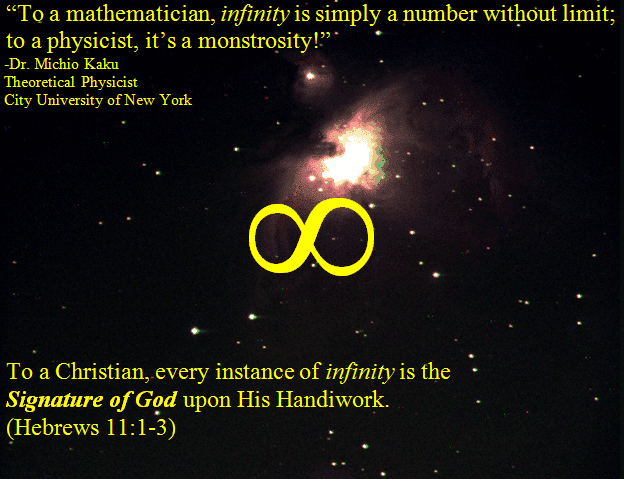‘Infinity’ is a very basic concept that God has built into the construct of His Creation. Most everyone understands what it implies; but, it is literally impossible to fully explain or even comprehend from a finite perspective.

This article takes a brief, common-sense approach to examining the concept of ‘infinity’ based on its mathematical definition in light of the Scriptures in order to demonstrate that wherever we find ‘infinity’ in nature (or the mathematics used to represent the physical universe) we are, in fact, viewing the ‘Signature of God’ upon His Creation.

Examining Infinity

In its most rudimentary form, the definition of ‘infinity’ includes the idea of something that is ‘without end’ – terms such as ‘boundless’ or ‘limitless’ are also often used when describing the concept of ‘infinity’. As we shall see in a moment, even these terms are woefully inadequate when attempting to define just what ‘infinity’ really is.

From a mathematical perspective, any value that is divided by ‘zero’ results in ‘infinity’. Mathematically we can represent this concept in the following manner: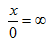Now, if this kind of mathematical notation scares you (please don’t be ashamed – I’ve taught many adults who are intimidated by math symbols and notations), don’t worry. Let’s put some actual NUMBERS in first so you can follow what’s going on as we move forward…

By definition, when a numerator (the ‘x’ in the previous formula) is divided by a denominator (the ‘0’ in the previous formula) to yield a result (the ‘∞’ in the previous formula); then, when the result is multiplied by the denominator it should return the numerator for an answer.

For Example: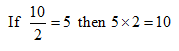In other words, the number in the denominator (bottom) of the fraction must be the correct number to multiply the result by in order to return the number in the numerator (top) of the fraction.

Returning now to our original expression, let’s first evaluate it by letting x=1: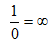Mathematically, this is a true statement which reads, “One divided by zero equals infinity.”

Now, as we have seen already, in order for this statement to be true, the following expression must also be true: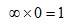What this means is that ‘infinity’ must represent some value that is so incredibly large that it can LITERALLY make ‘1’ out of ‘0’. In non-mathematical terms (although this is EXACTLY what the mathematics imply):

Infinity is a value that is capable of creating something out of nothing!!!

This sounds pretty incredible already – right?

It doesn’t end there, though…

Let’s returning once again to our original expression:We need to recognize that ‘x’ is just a ‘place-holder’ that can represent any finite number in the universe, up to and including an astronomical number that represents everything in the universe (and even more than that if we so choose)!!!

Nonetheless, the original expression STILL holds true, that ANY VALUE divided by zero yields ‘infinity’. This also means that the following expression is also true: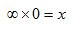So ‘infinity’ multiplied by ‘zero’ is capable of yielding any finite number no matter how large. This allows us to further modify the definition of ‘infinity’ as follows:

Infinity is a value that is capable of creating anything or everything out of nothing!!!

Using the strictest interpretation of the data that are presented to us by this simple mathematical expression the above definition of the capability of ‘infinity’ is completely valid.

Infinity and Creation

Atheistic mathematicians and physicists would love to do away with ‘infinity’ because, although they have no definition for its value, they must learn to contend with it because it is a REAL VALUE that is built into the very fundamentals of the mathematics we use. The reason why I specifically mention ‘atheists’ at this point is because this is where those who deny the existence of the Lord God as Creator of all things begin to look elsewhere for their answers. They abandon ‘infinity’ as ‘taboo’ and search for solutions that either deal ‘neatly’ with the concept of ‘division by zero’; or, try vehemently to turn away from solutions that result in ‘infinity’ altogether.

Conversely, through this simple mathematical expression, Christians have the opportunity to see the Signature of God upon all that He has created. Not only does the very mathematical definition of ‘infinity’ point directly to God as the Author of the mathematics that are used in the explanation of laws that govern our physical universe; but, the value of ‘infinity’ itself is encountered on a daily basis by everyone on planet earth.

Common irrational numbers such as π (pi) and e are among the many values representing real mathematical relationships in our physical world. These constant values are known as irrational numbers because their exact value cannot be calculated. This is also true of many numbers like ⅓ which as a decimal becomes 0.333333…. However, unlike the value of ⅓, which can be represented by an exact numerical or mathematical expression, both π and e cannot be represented in the manner – but only approximated. This kind of number is called a transcendental number.

While we have numerous mathematical formulas to approximate the values of π and e, even accurately giving us each next decimal point value in sequence, there is no actual pattern to the decimal values, and no sequence or sequences which repeat themselves. Therefore, no matter how many decimal places we calculate for these two numbers, we will NEVER know the next one until it has been calculated –AND– we will NEVER be able to find or represent the exact value of either number with absolute precision and accuracy! Therefore.

The determination of both π and e is a process of INFINITE CALCULATION.

So you may ask, why do we even look at π and e to begin with? What do they even mean anyway???

Of these two values, π is certainly the most familiar, and therefore the most commonly obvious. This value represents the ratio between the circumference and the diameter of a circle. In mathematical terms we would write it like this: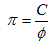where: C = circle circumference, ϕ = circle diameter

Simply put, if we divide the exact distance around the outside of a perfect circle by the distance across its diameter the result would be π.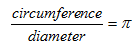This means that, even though the exact ratio of the circumference of a circle to its diameter exists in the physical world, we can NEVER exactly calculate that ratio because the value exists to an infinitely unknown number of decimal places!!!

The value of e is a bit more difficult to explain without going into extensive mathematical theory and detail; however, it is just as important (and almost as prevalent) as π in the physical world because it appears in so many formulas and equations that represent processes that take place in the natural world. Furthermore, like π the value of e exists to an infinitely unknown number of decimal places while representing a real and useable value in the physical world. Furthermore, each mathematical expression which accurately represents the value of e contains ‘∞’. Therefore, e (as is the case with virtually an infinite number of mathematical values) cannot exist without ∞. Thus it follows that:

Infinity is a necessary value without which our physical laws and the mathematics by which they are explained would be utterly unable to function.

Infinity and God

The Bible begins by telling us that “In the beginning God” created everything that is (Genesis 1:1). In the New Testament we further learn that ‘All things were made by Him and for Him’ and that ‘by Him all things consist’ – that is, they are held together (John 1:3; Colossians 1:16-17). Most importantly, however, we not only learn from the Bible that God has created everything that exists; but, that He has accomplished this so that, ‘Things which are seen were not made of things which do appear’ (Hebrews 11:3). This is a clear account of God not only creating ‘something from nothing’, but creating everything from nothing as well – an act in perfect harmony with the mathematical and physical definition of ‘infinity’.

We also learn according to the Bible, that God is possessed of three infinite properties. He is (1) Omniscient – all-knowing, (2) Omnipotent – all-powerful and (3) Omnipresent – everywhere at once and at all times.

Just a few of the many passages from the Scriptures that speak of these traits of God are listed below:

• Omniscient – ‘Thou knowest all things.’ (John 16:30 & John 21:17)
• Omnipotent – ‘Is there anything too hard for the Lord?’ (Genesis 18:14; Jeremiah 32:27)
• Omnipresent – His going forth is everywhere (Psalm 19:6). He is in all places at once (Psalm 139:7-8).

Therefore, not only does the work of the Lord point to an Infinite Creator; but, the Creator Himself by His very Nature is presented as Infinite in the Scriptures.

This is only fitting because by definition,

God must be Infinite in order to perform the work the Bible claims on His behalf.

Two great servants of the Lord attest to this truth in the Scriptures, one in the New Testament, and one in the Old.

In 1 Timothy 1:17 of the New Testament the Apostle Paul calls Him the ‘Eternal, Immortal, Invisible, and ONLY Wise God’ as he writes instructions on Church leadership to young Timotheus.

Moses writes in Psalm 90:2, ‘From everlasting to everlasting ([←∞ to ∞→] – eternity past to eternity to come) Thou art God’. This verse is especially revealing because it speaks of the Lord’s Eternal Nature as being without beginning and without end which is truly boundless.

Summary

We began our examination of the concept of infinity by stating that it is a concept that is easily understood from a general perspective, but is a concept that is utterly impossible to fully comprehend. The Scriptures teach us the very same thing about the Lord God who is the Creator of the Heavens and the Earth, when they record that, ‘His thoughts are above our thoughts’ and ‘His ways above our ways’. His Word tells us that, ‘His ways are past finding out’.

When we examine God from our perspective apart from the wisdom of His Word we are truly the finite attempting to explain the Infinite. The answers to all we need to know about Him are found in His Word. The Eternal Blessing we have been promised if we accept His Dear Son Christ Jesus as Savior, making Him Lord of our life, is that ‘one day we shall be like Him for we shall see Him as He is’!!!

## Written by Dr. Jack Burton

Dr. Burton has attended the United States Naval Academy in Annapolis, MD and is now retired from being employed as a professional in the field of Commercial Mechanical Engineering for more than 2 decades.
His professional areas of specialty include: air flow, fluid flow, and heat and mass transfer.

He is also a former Certified LEED (Leadership in Energy and Environmental Design) Professional specializing in building design and construction.

He has been awarded an Honorary Doctorate from Evangel Christian University of America for service in local ministry and work done in area of Biblical Doctrines.

As the founder of Noah’s Wonders resource ministry he now provides Bible Commentaries, resources, and references for the Foundational Truth of God's Word.

1. Nonetheless, the original expression STILL holds true, that ANY VALUE divided by zero yields ‘infinity’. This also means that the following expression is also true:

x/0 = inf implies (inf) * 0 = x

So ‘infinity’ multiplied by ‘zero’ is capable of yielding any finite number no matter how large. This allows us to further modify the definition of ‘infinity’ as follows:

Infinity is a value that is capable of creating anything or everything out of nothing!!!
_______________________________________________________________

An interesting thought but I don’t believe your mathematical reasoning is correct. Mathematically, any real quantity, ‘X’ divided by zero, is undefined. There is no other mathematical conclusion.

see Wolfram on this: “Zero does not have a multiplicative inverse under any circumstances.”

2. Charles,

In the sense that there is no ‘absolute’ value that satisfies the multiplicative inverse of zero, Wolfram (along with all of my math teachers & professors) is absolutely correct!!!

However, infinity (∞) is not used to represent any absolute value in mathematics or physics. We use the term ‘undefined’ or other similar expressions interchangeably with the ‘value’ of infinity – not to represent an ‘absolute’ value of any sort – but to represent a concept. Infinity by its very nature cannot be defined as an ‘absolute’ (or negative of an ‘absolute’ value) since it represents an ever-expanding quantity growing at an ever-increasing rate.

The true nature of infinity is seen in mathematics when we look at the finite values which can be determined in ‘determinate’ infinite series progressions by applying the laws of limits that converge in such a way that the final result without the use of those laws becomes undefined (such as 1/0, x/0, 0/0, etc.). This principle is discussed in detail by William A. Granville in ‘Elements of the Differential and Integral Calculus’, 2nd Edition, Chapter III: Theory of Limits.

Please let me know if this has provided clarification for you.

In His Love,
Dr. Jack L. Burton
-Hebrews 11:1-3

3. Dr. Burton,

This is an interesting article. One thing I don’t understand is: what exactly are you doing when you divide by zero? Take four pennies. If you divide by two, you get two piles of two each. If you divide by one, you get one pile of four. But how can you divide by zero, and how can it be different from dividing by one? Maybe I’m just being too practical.

Having said that, I have often thought it interesting that the naturalists cannot mathematically describe the creation of the universe: Zero does not equal universe. But with an infinite God, the equation balances: Infinity plus zero = infinity plus universe.

• Tim,
Indeed naturalists do find it quite a challenge to explain the origins of our universe – especially with all of its order and intricacies.

If course, my desire (and I hope your is as well), is not to ridicule the unbeliever. It is my sincere hope that by demonstrating the glory of our Lord’s Handiwork throughout His Creation, I may be used of Him to lead many who are lost to Christ.

Now, regarding your comments on division by ‘zero’ and ‘one’, here is a simple explanation of why division by ‘zero’ can be used to represent an infinite value:

First, we need to clarify the relationship between the mathematical operations of multiplication and division. These are known as ‘inverse’ operations. This means that we should be able to use the same set of values to perform a proper operation in both directions.

For example, if we divide 10 by 2 the result is 5.

10 ÷ 2 = 5

By using the SAME numbers we can multiply the ‘result’ (5) by the ‘dividend’ (2) and our new result should be the original ‘divisor’ (10).

5 × 2 = 10

Therefore, whenever we divide one number by another number we should be able to multiply the result by the number we divided by to get back the original number.

This works with all values EXCEPT ‘zero’.

By definition zero means ‘nothing’ and has no numerical value. So, no matter what we multiply zero by it will ALWAYS remain ZERO.

This is where the ‘concept’ of infinity comes in. We must understand that infinity is NOT a numerical value. Rather, it represents the concept of a value increasing so rapidly that it is somehow able to ‘push’ nothing (zero) into something (represented by 1 in the article).

So we begin with the following mathematical expression:

‘infinity’ x 0 = 1

This does not mean that ‘infinity’ multiplied by ‘zero’ literally equals the value of ‘1’. Rather, it is a representation that is meant to illustrate that an unattainable value is needed to ‘push’ zero (nothing) into having a value.

You can also easily satisfy yourself that division by ‘1’ and division by ‘0’ yield entirely different results by performing the following simple exercise on a calculator:

Enter ‘1’ then divide it by ‘1’.

1 ÷ 1 = 1

Next, enter ‘1’ then divide it by 1/10 (or ‘0.1’).

1 ÷ 0.1 = 10

By continuing this process you will see that as you divide ‘1’ by smaller and smaller values, the result will grow larger and larger (a few more values in this progression are listed below).

1 ÷ 0.01 = 100
1 ÷ 0.001 = 1,000
1 ÷ 0.0001 = 10, 000
.
.
.
1 ÷ 10^(-50) = 10^50
(‘1’ followed by 50 ‘zeroes’)

You can keep entering values as small as your calculator will allow & the result will keep getting larger. However, if you try to divide ‘1’ (or any other value) by ‘0’ on a calculator you will receive an error message. This is because there is no mathematical value that will satisfy the necessary criteria for the result (which by definition must be infinite).

I hope this has helped to clarify the concept of ‘division by zero’ and gives you a better understanding of the foundation for this article.

In Christ’s Love,
Jack B.
-Heb. 11:1-3